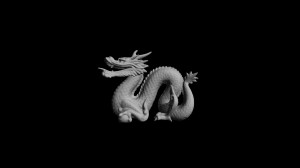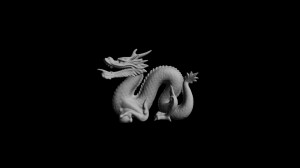# A preliminary Wavefront OBJ loader in C++

This post will examine the Wavefront OBJ file format and present a preliminary loader in C++. We will overlook the format's support for materials and focus purely on the geometry. Once our model has been loaded, an OpenGL Display List will be used to render the model. Below is a rendering of a dragon model available at The Stanford 3D Scanning Repository. In the OBJ file used for this render the vertex normals were not present. At run time, normals were evaluated at each face for lighting calculations yielding a flat shading.This is a rendering of the dragon without normals supplied in the OBJ file. Normals were evaluate for each face at run time.

In the render below we had imported the OBJ file into Blender, applied a smooth rendering, and exported the smoothed model. We then had a normal for each vertex allowing us to interpolate the normals across the face yielding a smooth shading. One way this could be done in our loader would be to assign a normal to each vertex, then for each face that uses a vertex, add that face's normal to the vertex normal, and, finally, ensure the vertex normal has unit length.This is a rendering of the dragon model after being passed through Blender. Normals were evaluated at the vertices for interpolation across the face at run time.

Below is a video capture of the smoothed dragon model at run time.

We will be parsing the OBJ file for vertices, normals, and faces. The texture coordinates and parameter vertices will also be parsed, but these will go unused for the time being.

A vertex definition will have the following forms where "v" is literal.

v x y z v x y z w 

Normals will have the following form where "vn" is literal.

vn i j k 

Finally, faces will have one of the following forms where "f" is literal.

f v0 v1 v2 ... f v0/vt0 v1/vt1 v2/vt2 ... f v0//vn0 v1//vn1 v2//vn2 ... f v0/vt0/vn0 v1/vt1/vn1 v2/vt2/vn2 ... 

In the first case only vertices are supplied, in the second we have vertices and texture coordinates, in the third we have vertices and normals, and, lastly, we have vertices, texture coordinates, and normals.

Below we define a couple of structures. The first is for a vertex which we will use for the vertices and normals (in addition to the texture coordinates and the parameter vertices). We have added a normalize method to ensure our normal vectors have unit length. The minus operator has been overloaded and a cross method added for evaluating face normals when normals have not been specified in the OBJ file. The second structure is to store the vertices, normals, and texture coordinates for a face.

struct vertex {
std::vector<float> v;
void normalize() {
float magnitude = 0.0f;
for (int i = 0; i < v.size(); i++)
magnitude += pow(v[i], 2.0f);
magnitude = sqrt(magnitude);
for (int i = 0; i < v.size(); i++)
v[i] /= magnitude;
}
vertex operator-(vertex v2) {
vertex v3;
if (v.size() != v2.v.size()) {
v3.v.push_back(0.0f);
v3.v.push_back(0.0f);
v3.v.push_back(0.0f);
} else {
for (int i = 0; i < v.size(); i++)
v3.v.push_back(v[i] - v2.v[i]);
}
return v3;
}
vertex cross(vertex v2) {
vertex v3;
if (v.size() != 3 || v2.v.size() != 3) {
v3.v.push_back(0.0f);
v3.v.push_back(0.0f);
v3.v.push_back(0.0f);
} else {
v3.v.push_back(v*v2.v-v*v2.v);
v3.v.push_back(v*v2.v-v*v2.v);
v3.v.push_back(v*v2.v-v*v2.v);
}
return v3;
}
};

struct face {
std::vector<int> vertex;
std::vector<int> texture;
std::vector<int> normal;
};


Below is the declaration of our cObj object for loading and rendering the model.

class cObj {
private:
std::vector<vertex> vertices;
std::vector<vertex> texcoords;
std::vector<vertex> normals;
std::vector<vertex> parameters;
std::vector<face> faces;
GLuint list;
protected:
public:
cObj(std::string filename);
~cObj();
void compileList();
void render();
};


The constructor will attempt to open the OBJ file for reading and populate the vertices, normals, and faces. Afterward, it calls the compileList method to generate the OpenGL Display List and then releases the memory allocated for the vertices, normals, and faces.

cObj::cObj(std::string filename) {
std::ifstream ifs(filename.c_str(), std::ifstream::in);
std::string line, key;
while (ifs.good() && !ifs.eof() && std::getline(ifs, line)) {
key = "";
std::stringstream stringstream(line);
stringstream >> key >> std::ws;

if (key == "v") { // vertex
vertex v; float x;
while (!stringstream.eof()) {
stringstream >> x >> std::ws;
v.v.push_back(x);
}
vertices.push_back(v);
} else if (key == "vp") { // parameter
vertex v; float x;
while (!stringstream.eof()) {
stringstream >> x >> std::ws;
v.v.push_back(x);
}
parameters.push_back(v);
} else if (key == "vt") { // texture coordinate
vertex v; float x;
while (!stringstream.eof()) {
stringstream >> x >> std::ws;
v.v.push_back(x);
}
texcoords.push_back(v);
} else if (key == "vn") { // normal
vertex v; float x;
while (!stringstream.eof()) {
stringstream >> x >> std::ws;
v.v.push_back(x);
}
v.normalize();
normals.push_back(v);
} else if (key == "f") { // face
face f; int v, t, n;
while (!stringstream.eof()) {
stringstream >> v >> std::ws;
f.vertex.push_back(v-1);
if (stringstream.peek() == '/') {
stringstream.get();
if (stringstream.peek() == '/') {
stringstream.get();
stringstream >> n >> std::ws;
f.normal.push_back(n-1);
} else {
stringstream >> t >> std::ws;
f.texture.push_back(t-1);
if (stringstream.peek() == '/') {
stringstream.get();
stringstream >> n >> std::ws;
f.normal.push_back(n-1);
}
}
}
}
faces.push_back(f);
} else {

}
}
ifs.close();
std::cout << "               Name: " << filename << std::endl;
std::cout << "           Vertices: " << number_format(vertices.size()) << std::endl;
std::cout << "         Parameters: " << number_format(parameters.size()) << std::endl;
std::cout << "Texture Coordinates: " << number_format(texcoords.size()) << std::endl;
std::cout << "            Normals: " << number_format(normals.size()) << std::endl;
std::cout << "              Faces: " << number_format(faces.size()) << std::endl << std::endl;
list = glGenLists(1);
compileList();
vertices.clear();
texcoords.clear();
normals.clear();
faces.clear();
}


The compileList method builds the OpenGL Display List. This method expects the faces to be either triangles or quadrilaterals. If normals were specified in the OBJ file, they are used here; otherwise, a normal is evaluated at each face.

void cObj::compileList() {
glNewList(list, GL_COMPILE);
for (int i = 0; i < faces.size(); i++) {
if (faces[i].vertex.size() == 3) { // triangle
if (faces[i].normal.size() == 3) { // with normals
glBegin(GL_TRIANGLES);
glNormal3f(normals[faces[i].normal].v, normals[faces[i].normal].v, normals[faces[i].normal].v);
glVertex3f(vertices[faces[i].vertex].v, vertices[faces[i].vertex].v, vertices[faces[i].vertex].v);
glNormal3f(normals[faces[i].normal].v, normals[faces[i].normal].v, normals[faces[i].normal].v);
glVertex3f(vertices[faces[i].vertex].v, vertices[faces[i].vertex].v, vertices[faces[i].vertex].v);
glNormal3f(normals[faces[i].normal].v, normals[faces[i].normal].v, normals[faces[i].normal].v);
glVertex3f(vertices[faces[i].vertex].v, vertices[faces[i].vertex].v, vertices[faces[i].vertex].v);
glEnd();
} else { // without normals -- evaluate normal on triangle
vertex v = (vertices[faces[i].vertex] - vertices[faces[i].vertex]).cross(vertices[faces[i].vertex] - vertices[faces[i].vertex]);
v.normalize();
glBegin(GL_TRIANGLES);
glNormal3f(v.v, v.v, v.v);
glVertex3f(vertices[faces[i].vertex].v, vertices[faces[i].vertex].v, vertices[faces[i].vertex].v);
glVertex3f(vertices[faces[i].vertex].v, vertices[faces[i].vertex].v, vertices[faces[i].vertex].v);
glVertex3f(vertices[faces[i].vertex].v, vertices[faces[i].vertex].v, vertices[faces[i].vertex].v);
glEnd();
}
} else if (faces[i].vertex.size() == 4) { // quad
if (faces[i].normal.size() == 4) { // with normals
glNormal3f(normals[faces[i].normal].v, normals[faces[i].normal].v, normals[faces[i].normal].v);
glVertex3f(vertices[faces[i].vertex].v, vertices[faces[i].vertex].v, vertices[faces[i].vertex].v);
glNormal3f(normals[faces[i].normal].v, normals[faces[i].normal].v, normals[faces[i].normal].v);
glVertex3f(vertices[faces[i].vertex].v, vertices[faces[i].vertex].v, vertices[faces[i].vertex].v);
glNormal3f(normals[faces[i].normal].v, normals[faces[i].normal].v, normals[faces[i].normal].v);
glVertex3f(vertices[faces[i].vertex].v, vertices[faces[i].vertex].v, vertices[faces[i].vertex].v);
glNormal3f(normals[faces[i].normal].v, normals[faces[i].normal].v, normals[faces[i].normal].v);
glVertex3f(vertices[faces[i].vertex].v, vertices[faces[i].vertex].v, vertices[faces[i].vertex].v);
glEnd();
} else { // without normals -- evaluate normal on quad
vertex v = (vertices[faces[i].vertex] - vertices[faces[i].vertex]).cross(vertices[faces[i].vertex] - vertices[faces[i].vertex]);
v.normalize();
glNormal3f(v.v, v.v, v.v);
glVertex3f(vertices[faces[i].vertex].v, vertices[faces[i].vertex].v, vertices[faces[i].vertex].v);
glVertex3f(vertices[faces[i].vertex].v, vertices[faces[i].vertex].v, vertices[faces[i].vertex].v);
glVertex3f(vertices[faces[i].vertex].v, vertices[faces[i].vertex].v, vertices[faces[i].vertex].v);
glVertex3f(vertices[faces[i].vertex].v, vertices[faces[i].vertex].v, vertices[faces[i].vertex].v);
glEnd();
}
}
}
glEndList();
}


Lastly, the render method calls the display list and the destructor releases it.

void cObj::render() {
glCallList(list);
}

cObj::~cObj() {
glDeleteLists(list, 1);
}


Instantiating the object should occur after an OpenGL context has been created.

	cObj obj("media/dragon_smooth.obj");


Rendering the object.

	obj.render();


We have not implemented much in the way of error checking or materials. Additionally, the OBJ file format has support for curves and surfaces. Hopefully, we can get around to implementing some of these other features, but for now download the project and modify it.